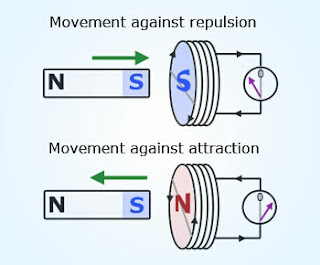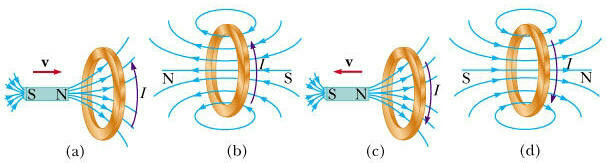# Lenz's Law

Views

## Lenz's Law

Introduction

Before I start  to write my  article about  Lenz's law  , it becomes necessary  for me  to provide you some information related to the person , who has discovered this amazing concept , which is of vital importance in the field of electromagnetism , physics.

This concept in physics was given by a well known Russian physicist Heinrich Friedrich Emil Lenz  in 1833, a fundamental law of electromagnetism. He also found that the strength of a magnetic field is proportional to the strength of the magnetic induction. This well known scientist was born and educated in Dorpat (now Tartu, Estonia).

Before switching to science , he studied theology. Lenz received doctor of philosophy degree , a common thing for scientists of that time. Writing as a naturalist, Lenz  travelled around the world , when he was just 20 years old. Lenz  presented his scientific papers at the Imperial Academy of Sciences at St. Petersburg.

There is a common way to understand, how electromagnetic circuits obey law of conservation of energy and Newton's third law,  and that's  Lenz's law . It is named after Emil Lenz  which states that;

### Statement of  Lenz's Law :

Lenz's law  states that the direction of induced EMF  is such that, it opposes the cause which is responsible for its production.

What is Induced EMF ?

We can say induced EMF  as the production of  electromotive force that's a voltage across an electric conductor in a changing magnetic field .
Faraday  used the concept  called " lines of force " and explained electromagnetic induction . However, these theoretical ideas of Faraday were widely rejected by the scientists at the time, because there was no mathematical formula presented by Faraday at the time.

#### step1:

For the verification of  Lenz's law , it is important for us to have essential materials like galvanometer,  two way key, a battery , magnet and a coil.Experimental verification of Lenz's law

#### step2:

Take a coil AB. Connect a galvanometer G, a two way key  K and a battery C between terminals A and B of the coil.

#### Step3:

Connect the terminals 1 and 2 of the two way key. Due to this, battery C sends current in the anti - clockwise direction.

After this event, the upper face of the coil which is denoted by A becomes N-pole. Suppose in this case, we observe that the galvanometer gives deflection towards left.

Hence, we conclude that when the galvanometer gives the deflection towards left position, the upper face 'A' of the coil becomes N-pole and vice versa.

#### Step 4:

After the completion of the process by the terminals 1 and 2  , we need to disconnect them. Now connect the terminals 2 and 3 of the two way key.  Take a magnet  denoted by NS with its north pole towards the coil.  Now, when we start moving the magnet towards the coil, we note that the galvanometer gives us deflection towards the left position. Hence the Upper face of the coil denoted by A becomes N-pole. Hence, now the north pole of the coil repels the north pole of the magnet

Hence, we can observe that the direction of induced EMF opposes the inward motion (cause)  of the magnet.

#### step 5:

Similarly, we can also verify that the direction of the induced EMF  also opposes the outwards motion of the magnet.

#### step 6:

Thus ,we can say that the direction of the induced EMF is always such that it opposes the cause responsible for its production.

#### Lenz's Law and Energy conservation :

Lenz's law  is consistent with the Principle of law of Conservation of Energy because when a magnet with its N-pole facing  the coil is pushed toward the coil, there is a increase (or decrease) in magnetic flux linkage, resulting in an induced current flowing in the cell, which happens according to Faraday's Law. Below it is explained in an exact way.

When the north pole of the magnet is moved towards the coil, the upper face of the coil, which is denoted by A  becomes North Pole. Now this north pole of the coil starts to repel the north pole of the magnet . Therefore work is to be done in moving the magnet towards the coil.Lenz's law and energy conservation

When N-pole of the magnet is moved away from the coil, the upper face of the coil becomes S-pole .The S-pole of the coil starts to attract the north pole of the magnet . Therefore, some amount of work is to be done in moving the magnet away from the coil. This work done is converted into the electrical energy.

Thus, in the conclusion I becomes clear for us that the  Lenz's law  obeys the principle of conservation of energy.

Note:

It should be noted that Michael Faraday, a well known physicist is credited with the discovery of induction in the year 1831 . After this another scientist named James clerk Maxwell mathematically described it as Faraday's law's of electromagnetic induction. The direction of the induced EMF was later described by the  Lenz's law . There are many applications of electromagnetic induction like transformers, generators ,inductors , current clamp, electromagnetic forming, induction cooking, hall effect meters,  inductors, magnetic flow meters Rowland ring, wireless energy transfer and electric motors.

Conclusion

The article has discussed the concept of  Lenz's law  and energy conservation. The article also explains you the experimental verification of  Lenz's law. It was basically described to show the direction of induced EMF. The concept of induced EMF is also described in the report. The article also aware you about Heinrich  Lenz, one among the famous scientists. The article has discussed completely about Lenz , his law and energy conservation . I Hope ,this article would have helped you a lot in knowing this concept. Please share it to your friends and others, so that they can also get the benefits of the same.